Kaleidocycles
 To the Main Page     "Mathematische Basteleien"

What is a Kaleidocycle?
 ...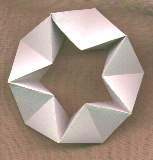... K1 The simplest kaleidocycle is a ring of an even number of tetrahedra. (Tetrahedra are pyramids with equal edges and equilateral triangles as sides.) The tetrahedra are connected on perpendicular edges.  What makes it special is that you can continually twist it inwards or outwards while it shows different sides of each tetrahedron.
There is an infinite number of kaleidocycles. The tetrahedra becomes a pyramid then.

On this page I introduce and describe special kaleidocycles. I number them with K1 to K15.

The Ring of Eight Tetrahedra top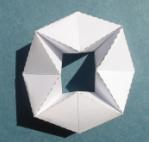K2

The smallest amount is eight tetrahedra.
 ...... The drawing on the left shows a position, where the joint edges are horizontal or vertical.  In the drawing on the right you see a cross-section of this model. a is the edge of a tetrahedron, h=sqrt(3)/2*a is the altitude of an equilateral triangle.  The square with the broken line is the foundation of the ring. ......

Making of the Ring
You can produce the ring with paper.

You can make the eight tetrahedra separately and connect them with adhesive tape. This is simple, but long work.

It is more challenging to design a net of the ring, but folding it is a bit more difficult.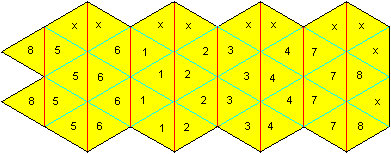1) Draw the net with equilateral triangles with compasses and a ruler. The numbers 1 to 8 are only for explaining. The x indicates the surfaces to be glued together.
The length of a triangle is e.g. 2.5 cm; then the drawing fits an A4 page.
(2) Cut out the net.
(3) Go over the lines with a ballpen or the blunt edge of scissors, so that you can fold the paper at the lines more easily. Fold the paper several times.
(4) First form the tetrahedra 1111 and 2222 at the same time. Glue the triangles xx (top) on the triangles 12 (down).
(5) Repeat the process with the pairs 3333/4444, 5555/6666 and 7777/8x8x in this order.
(6) Close the ring by gluing the triangles 88 on xx last.

If you want to make rings with 10,12,14, ... tetrahedra,  put in more strips.

Closed Ring of Six Pyramids     top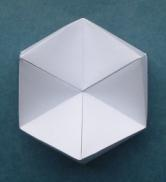K3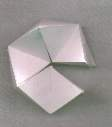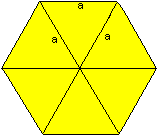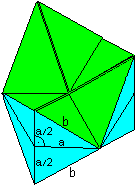If you try to assemble six tetrahedra to a ring, you see that they don't form a ring (picture on the left). You must stretch the tetrahedra, so that you achieve a ring. In the borderline case you have a hexagon as a cross-section (picture in the middle). On the right you can see the ring.
There is a right angled triangle with the sides b, a and a/2. The Pythagorean theorem gives  b²=a²+(a/2)² or b=sqrt(5)/2*a.

You have 4-sided pyramids instead of tetrahedra.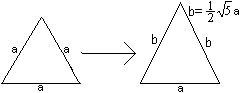... The triangles of the tetrahedra are no longer equilateral but isosceles.  The ratio of one side to the other one is b : a = 1.12 : 1.
Template:M.C.Escher Kaleidocycles top
 ..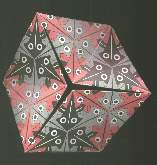... In the book (1) below, the ring with stretched tetrahedra is called kaleidocycle. This name is generally accepted because of the world-wide spread of this book.  The book has 14 models and instructions, among them several rings.  The models are decorated with parts of the paintings of the Dutchman M.C.Escher. So they become nice and unique.  A closed kaleidocycle is fascinating, because there is a lot of  movement during rotation. The middle is opened and closed regularly.

Closed Ring of Eight Pyramids   top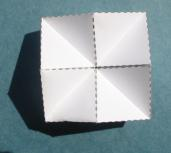K4
 ...... If the ring of eight tetrahedra from above shouldn't have a hole, you must change the shape. That leads to a square as a cross-section. You must imagine that there are edges perpendicular to the drawing-board going through the middles of the sides. P is one centre point.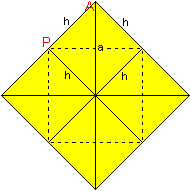......

 ...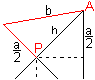...E If you turn the vertical edge a in P in the drawing board, you get a right angled triangle.  You can calculate the length of the edge b of the pyramid:  b²=(a/2)²+h².  You get  b=[sqrt(3)/2]a with h=sqrt(2)/2*a.

You have 4-sided pyramids instead of tetrahedra. The triangles are no longer equilateral but isosceles.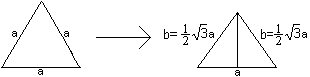The ratio of one side to the other one is b : a = 0,87 : 1.

Template:Origin: Randolf Rehfeld

The Invertible Cube top

...K5
How to make it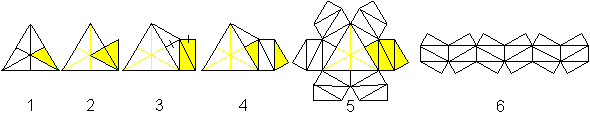(1) Draw an equilateral triangle and its medians (a=5cm for example).
(2) Reflect a triangle on a side.
(3) Erect a rectangle on the hypotenuse of the reflected triangle. The marked lines are equal.
(4) Put  the triangle on the rectangle.
(5) Repeat this process five times. Fold a pyramid of the four yellow right angled triangles.
(6) Form a row and add strips on the triangles to fix the pyramids and to close the ring later.

Result: You get a kaleidocycle of six pyramids with a triangular hole.

Template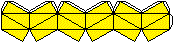Description
 ...... There are four positions remarkable while twisting inwards:  (1) Six triangles of the pyramids lie in the plain (picture 1). They form a hollow hexagon in the front.  (2) You twist further. There is the same hexagon on the reverse side.  (3) Then the six triangles form an equilateral triangle on the reverse side.  (4) This triangle turns to the front side (picture 2).
This kaleidocycle is known as the invertible cube or Schatz cube.

To explain the name "invertible cube", you may think to look at the well known hexagon inside a cube. You can see the triangles inside and outside the hexagon, but I found no relation to the whole pyramids.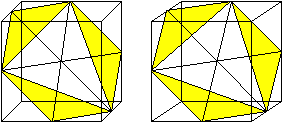If you can use the 3D-view, you recognize the six basic sides of the pyramids.

This explains the name "invertible cube":
You can turn the ring so that the right angles of the triangles are used to form corners of the cube: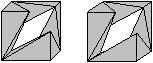You recognize the six pyramids of the ring. The cube is not solid. The pyramids only fill a third of the cube. You can prove it:

Calculation
 ...... A pyramid is formed by four triangles inside an equilateral triangle with the side a. A triangle has the sides a/2, h/3, and 2h/3. The height is h=[sqrt(3)/2]a. The length of the side of the cube is a/2 and thus the volume is V'=a³/8. The pyramids altogether have the volume 6*(1/3)*area of the triangle*height.
This is here V=6(1/3)[(1/2)(a/2)(h/3)][h/3]=(1/24)a³. Therefore is V=V'/3.

If you want to complete the cube as a solid cube, you need two equal "bolts" to put into the hollow cube.The Ring Half Octahedrontop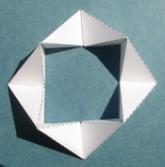K6
You can develop the invertible cube to a ring with an open square instead of an equilateral triangle using special positions.

How to make it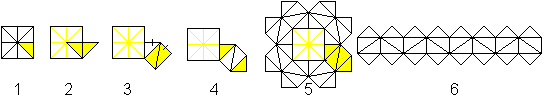(1) Draw a square with diagonals and its medians ( a=5cm for example).
(2) Reflect a triangle on a side.
(3) Erect a rectangle on the hypotenuse of the reflected triangle. The marked lines are equal.
(4) Put the triangle on the rectangle.
(5) Repeat this process seven times. Fold a pyramid of the four right triangles.
(6) Form a row and add strips on the triangles to fix the pyramids and to close the ring later.

TemplateThis ring is called half octahedron here, because you can fold this ring to a (red) half octahedron.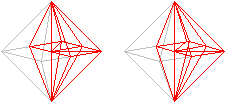Origin: Randolf Rehfeld (URL below) - There is a template.Double Crown  topK15
 ...... ...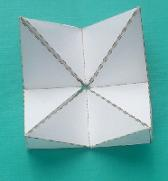... The kaleidocycle, which can be folded to a half octahedron, has a square window.  If you fold it further, you get a "crown" with four points. The base of this crown is a square.  Reflect it on the square as mirror.

 ...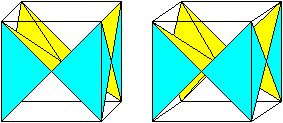... This leads to a new kaleidocycle, the "double crown". I didn't find a better name. You can recognize in this position that there is a relationship to a surrounding cube. Two triangular pyramids form an edge of the cube.  A calculation shows that the kaleidocycle is filling a third of the cube.

In this partition the cube is called Schneider-Würfel on Margarita Ehrlich's and Ellen Pawlowski's German web sites (URL below).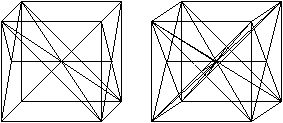transparent cube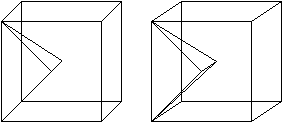one triangular pyramid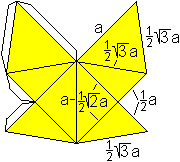4x Four "butterflies" can be used as templates (a=5cm). Each figure forms an edge of the corresponding cube.  The strips for glueing are missing on the right.

 ...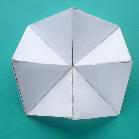... You can turn this kaleidocycle in that way that a crown with square base arises again.  The four points are lower.  You can again reflect this crown on a square and - voilá - you have a new kaleidocycle...

The Half Closed Ring of Six Pyramids   topK7

How to make this ring
 ...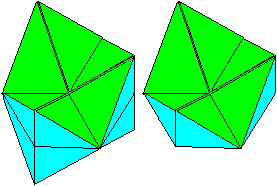... The closed ring of six pyramids from above has a symmetry plane. You can lay an intersection in it. A kaleidocycle develops which is erected over a regular hexagon.  It is special that the common edges are no longer the same.
If you twist the ring, you get two remarkable positions of the ring.The opening is an equilateral triangle (red). The shape is a non-regular hexagon with equal sides.The shape is an equilateral triangle (red).  The ring is nearly closed.
There is a symmetry plane in both cases (marked red).

Six squares and equilateral triangles on top form the net.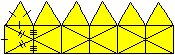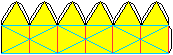The marked lines are the same. Those with one small stroke have the length a, with two strokes b=sqrt(5)/2*a and with three strokes a/2.

There is a similarity with the invertible cube described above.
In both cases there are positions with a triangle as silhouette. But it isn't closed as Schatz cube is. The edge a/2 is slightly shorter than sqrt(3)/3*a=0,56a at the Schatz cube.
This ring ought to be a hint to look for new kaleidocycles and to work on them.

You could already read above that there is an endless number of kaleidocycles.
A "normal" kaleidocycle is always formed if there are two opposite edges in pairs and a common perpendicular orthogonal.

The Shinsei Miracle    top
There is a golden/silver cube which was developped by Naoki Yoshimoto in the 1970s. You can take it apart and - a surprise! - you get two kaleidocycles of 12 pyramids.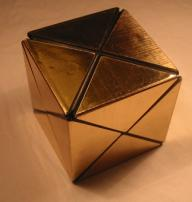----->K8K8
You can read more on my German page The Shinsei Miracle.

Cube One   top

Cube One is a new cube puzzle invented by the graphic designer and artist Dieter A.W. Junker from Kassel / Germany.

The challenge is to form a cube of  2x2 Kaleidocycles.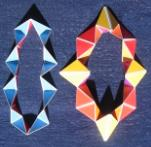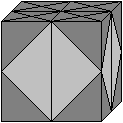Each kaleidocycle is a puzzle itsself.
One is leading to a tetrahedron, the other to an octahedron.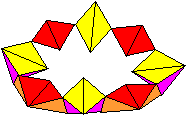K11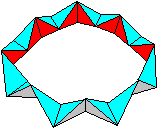K12You can find more on my page Cube One.

Tetra One   top
Tetra One is another puzzle developped by Dieter A.W. Junker.

The challenge is to form a tetrahedron with two Kaleidocycles. Both kaleidocycles only differ in the order of the pyramids.K13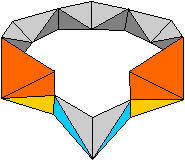K14You can find more on my page Tetra One.

Templates   top
You find descriptions on this page how to draw nets in order to build kaleidocycles.

But it is easier to use the templates which are available on the internet. I add the names Ki in my list of links and books, if you can find a template.

Have fun in building kaleidocycles and in looking for new shapes.

Kaleidocycles on the Internet    top

German

Franz Zahaurek
Der umstülpbare Würfel nach Paul Schatz

Randolf Rehfeld (Wundersames Sammelsurium)
Kaleidozyklen...K2, K3, K4, K5, K6

English

Akira Nishihara
Ring of tetrahedrons

Arvind Gupta
PAPER FLEXAGON ENGLISH 20MB     (Video at Youtube)

David Singmaster
Cubic Circular (magazine Issue 5 & 6 - #11 -  #13) (Autumn & Winter 1982)

Enchanted Learning online
Make A 3-D Hexaflexagon ...  K2

G. Korthals Altes
Paper Models of Polyhedra
Kaleidocycles:  Hexagonal Kaleidocycle K3, Octagonal Kaleidocycle K2, Decagonal Kaleidocycle K1

Herbert Kociemba
Kaleidocycles with 6 Disphenoids

instructables craft
Kaleidocycle

Jaap Scherphuis
Pyrix (Chain of 4 octahedrons + 11 tetrahedrons = 1 large tetrahedron)

Maurice STARCK
a ride through the polyhedra world

Kristina Burczyk's Kaleidocycles
Coloured Kaleidocycles with opened triangulars

Paul Jackson

How to Make a Kaleidocycle

Wikipedia
Kaleidocycle

French

Peuplier (Le Forum en Papier)
Kaleïdocycle didactique, le moteur 4 temps K4
Cube magique, Shinsei Mystery (ou Miracle) K8

References   top
(1) Doris Schattschneider und Wallace Walker, M.C.Escher Kaleidozyklen, Köln 1992  K3 K4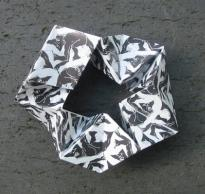The drilled kaleidocycle    K9

(2) Gerald Jenkins and Anne Wild, Make Shapes 1, Diss (Norfolk), Tarqin Publications, 1998   K1

Gail from Oregon Coast, thank you for supporting me in translating this website.

Feedback: Email address on my main page